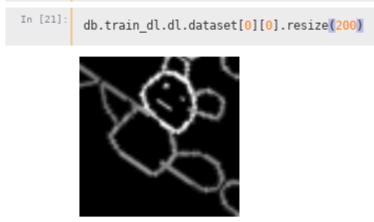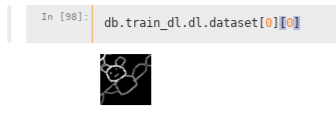# Unable to change padding to zeros when using rotation / warp from get_transforms()

I would like to use zeros padding. As I was not seeing a nice way of doing this when calling the following:

`db = ImageDataBunch.create(*datasets, path=PATH, bs=bs, ds_tfms=get_transforms(max_zoom=0, max_warp=0, max_rotate=0), size=sz)`

I tried making the changes directly in `vision/transform.py` but regardless what edits I make, I still get reflection padding both when applying rotate or warp.I also was unable to construct manually whatever get_transforms returns (maybe this way passing `mode='zeros'` or something along those lines to one of the transforms would work).

Any help on this would be greatly appreciatedThank you!

1 Like

I think `get_transforms` is more a convenience function, you will probably have to create a list of transforms yourself. You can see in the docs, that e.g. `crop_pad` has a `padding_mode` parameter that can be set to ‚zeros‘, the `pad` transform has the same but there it is simply named `mode`. The default of the padding parameter seems to be set to ‚reflection‘ everywhere.

2 Likes

Does it work if you pass padding_mode =‘zeros’ in `ImageDataBunch.create` ?

1 Like

Seems that get transforms returns a collection of transforms, the first element being transforms to be applied to the train set and the second to the val set.

Was getting an error earlier (probably due to autoreload) but now it seems I can construct the collection of transforms manually like so:

I still get same results though:The rotate function dos not accept any arguments regarding padding and it is very straightforwad:

``````def rotate(degrees:uniform):
"Rotate image by `degrees`."
angle = degrees * math.pi / 180
return [[cos(angle), -sin(angle), 0.],
[sin(angle),  cos(angle), 0.],
[0.        ,  0.        , 1.]]
``````

This, through the decorator, actually ends up being an instance of `Transform` (the function gets passed into the constructor). Now I don’t see anything in the `Transform` class that could be causing this behavior.

Could it be that when the 3x3 transformation from the rotate function gets applied to the gird, it automatically creates the reflection behavior?

The `padding_mode` should be passed to the `apply_tfms` function (which is where everything is done). Unless something is wrong, it’s when you create your data object you can pass it:
`data = ImageDataBunch.create(*datasets, path=PATH, bs=bs, ds_tfms=get_transforms(max_zoom=0, max_warp=0, max_rotate=0), size=sz, padding_mode='zeros')`

3 Likes

Hah, thats what I just found out from the code.But this `apply_tfms` is only used when passing in `ds_tfms`. What if we want to use ‘regular’ `tfms` that get used in the DataLoader? What is actually the difference between the two? And can we make the regular `tfms` also accept the padding parameter?

Thank you very much for your help SylvainWhen passing `padding_mode` to `get_transforms` I receive the following error:

I think I am on fastai version from a day or two ago, but will upgrade and see if I continue to get the same error msg.

You need to put the padding_mode outside of the get_transforms func, before/after size=sz I think. It needs to be a kwarg of the create method.

1 Like

Thank you very much for your help… and very sorry… I absolutely do not know how I missed this.

Works nowFor all the options, check the docs. We have kittens1 Like

I just wanted to share my solution when running into padding mode problem using SegmentationItemList class.
Here is my piece of code for databunch init:

``````codes = np.array(['item', 'background'])

data = (SegmentationItemList.from_folder(path_img)
.split_none()
.transform([tfms, None], tfm_y=True, size=500, resize_method=2, padding_mode='border')`
.databunch(bs=4)
)
``````

where my transformations are defined as

``````max_rotate = 180
max_warp = 0.2
max_zoom = 1.1
max_lighting = 0.5
p_affine = 0.75
p_lighting = 0.5

crop(size=500),
rotate(degrees=(-max_rotate, max_rotate), p=p_affine),
symmetric_warp(magnitude=(-max_warp,max_warp), p=p_affine, invert=False),
rand_zoom(scale=(1.,max_zoom), p=p_affine),
brightness(change=(0.5*(1-max_lighting), 0.5*(1+max_lighting)), p=p_lighting, use_on_y=False),
contrast(scale=(1-max_lighting, 1/(1-max_lighting)), p=p_lighting, use_on_y=False)]
``````

Despite having set padding mode to `border` in pad and .transform I kept getting `reflection` padding which lead to incorrect scraps of items scattered around corners after transformations.

The solution to this behavior was modifying the order of transformations for rand_zoom and symmetric_warp from 4 and 5 to 11.

``````tfms.tfm.order = 11
tfms.tfm.order = 11
``````

I didn’t find any information in the docs about the effect of transformation order on padding.

#Edit
After digging into it I found that this behaviour might be coming from here

``````def _image_maybe_add_crop_pad(img, tfms):
tfm_names = [tfm.__name__ for tfm in tfms]
``````

``````if resize_method <= 2 and size is not None: tfms = self._maybe_add_crop_pad(tfms)